Next: Real Cameras Up: The Perspective Camera Previous: The Perspective Camera

## Perspective Projection

Following Dürer and the Renaissance painters, perspective projection can be defined as follows (see fig. 1.3). The center of projection is at the origin O of the 3D reference frame of the space. The image plane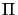is parallel to the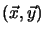plane and displaced a distance f (focal length) along the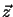axis from the origin. The 3D point P projects to the image point p. The orthogonal projection of O ontois the principal point o, and theaxis which corresponds to this projection line is the principal axis (sometimes called the optical axis by computer vision people, although there is no optic here at all).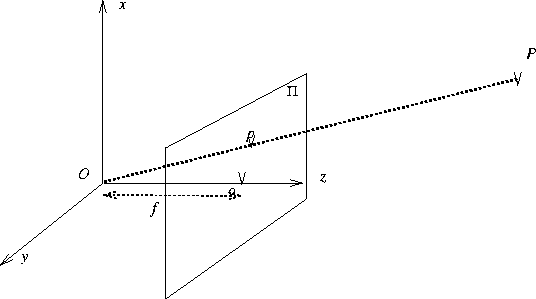Let (x, y) be the 2D coordinates of p and (X, Y, Z) the 3D coordinates of P. A direct application of Thales theorem shows that: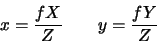We can assume that f=1 as different values of f just correspond to different scalings of the image. Below, we will incorporate a full camera calibration into the model. In homogeneous coordinates, the above equations become: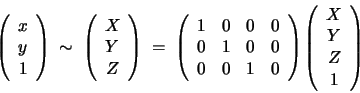In real images, the origin of the image coordinates is not the principal point and the scaling along each image axis is different, so the image coordinates undergo a further transformation described by some matrix K. Also, the world coordinate system does not usually coincide with the perspective reference frame, so the 3D coordinates undergo a Euclidean motion described by some matrix M (see exercise 1.3), and finally we have: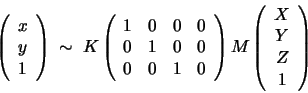(1.1)

M gives the 3D position and pose of the camera and therefore has six degrees of freedom which represent the exterior (or extrinsic) camera parameters. In a minimal parametrization, M has the standard 6 degrees of freedom of a rigid motion. K is independent of the camera position. It contains the interior (or intrinsic) parameters of the camera. It is usually represented as an upper triangular matrix: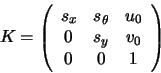(1.2)

where sx and sy stand for the scalings along the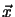and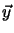axes of the image plane,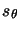gives the skew (non-orthogonality) between the axes (usually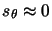), and (u0, v0) are the coordinates of the principal point (the intersection of the principal axis and the image plane).

Note that in homogeneous coordinates, the perspective projection model is described by linear equations: an extremely useful property for a mathematical model.Next: Real Cameras Up: The Perspective Camera Previous: The Perspective Camera
Bill Triggs
1998-11-13